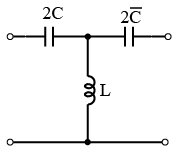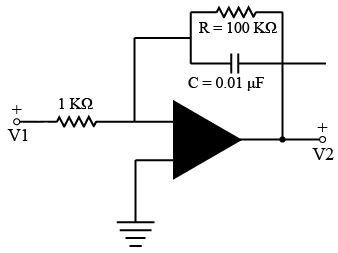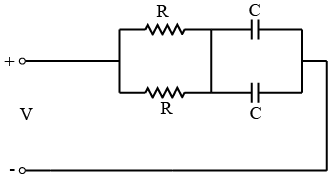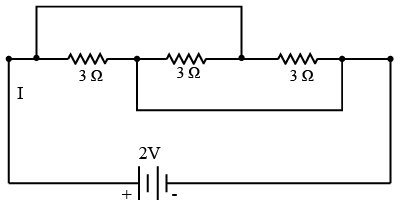# ISRO Scientist or Engineer Electronics 2006

Instructions

For the following questions answer them individually

Question 71

# Which loss in a transformer varies significantly with load?Question 72

# The resistance of a parallel circuit consisting of two resistors is 12 Ω One of the resistance wires breaks and the effective resistance becomes 18Ω. The resistance of the broken wire isQuestion 73

# Time constant of a Series R-L circuit equalsQuestion 74

# A dc voltage V is applied at time t = 0 to a series RC circuit. The steady state current isQuestion 75

# The given circuit represents aQuestion 76

# The very low frequency gain of the low pass filter shown in the given figure isQuestion 77

# The time-constant of the network Shown in the figure isQuestion 78

# For the circuit shown below the current l flowing through the circuit will beQuestion 79

# When L is doubled and is halved, the resonance frequency of series tuned circuit becomesQuestion 80

# In a Series resonant circuit, with the increase in LOR